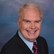# Math Solutions: Flow Rate ConversionsBy Daniel Theobald, "Wastewater Dan"

Water Online’s “Math Solutions” series, presented by wastewater consultant and trainer Dan Theobald (“Wastewater Dan”), provides an understanding of the sometimes difficult calculations involved in achieving wastewater operator certification.

Flow data is provided in many units of expression, such as liters or milliliters in the metric system, or gallons in an American system. It may also be provided in many different time increments, including seconds, minutes, hours, or days.

Depending on your calculation using flow, converting the unit or time of expression may be required for an accurate calculation.

Some common flow rate conversion calculations are accessible below:

Calculate/Convert Between Cubic Meters And Gallons

Cubic meters per second (cms) to million gallons per day (MGD):

Calculate/Convert Between Cubic Feet And Gallons

Cubic feet per second (cfs) to million gallons per day (MGD):

Calculate/Convert Between American Used System And Metric System

Gallons per minute (GPM) to liters per minute (L/min):

Calculate/Convert Flow Within The American System

Gallons per minute (GPM) to gallons per day (GPD):